Courses
Courses for Kids
Free study material
Offline Centres
More

# Word Problems on 4 Digit Addition and SubtractionLast updated date: 02nd Dec 2023
Total views: 99.6k
Views today: 2.99k## Introduction

Hey kids! Do you know the difference between addition and subtraction?

Addition means combining all the numbers, whereas subtraction is the difference between the numbers. To successfully perform any 4-Digit Addition and Subtraction, first, we need to know the addition and subtraction.

Now, we know what four-digit addition and subtraction are. It is the process of adding and subtracting two or more numbers with four digits each. The result is the difference between the four numbers or their sum.

To find a sum, an addition and an addend are added. The amount can also be referred to as the "total." A minuend is the beginning number in a subtraction operation and the number from which we subtract another integer.

Today's discussion will focus on 4-digit addition and subtraction word problems.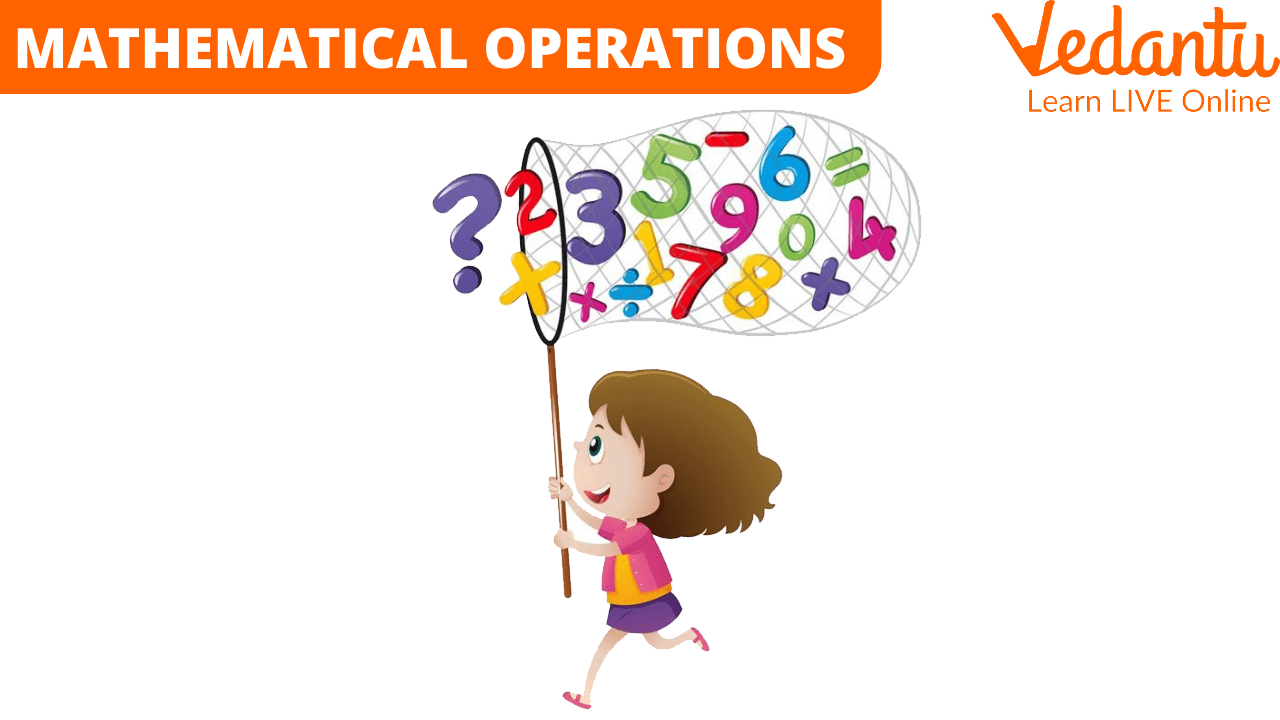Mathematical operations

Adding two or more numbers together is known as an addition in mathematics. The terms addends and total refer to the numbers added and the operation's result. We use number addition in a variety of situations. When we work with time or money, such as bills and receipts, adding numbers is one of daily life's most frequently used operations.

For example, when we see “2 + 1”, we read it as “two plus one”.

## What is Subtraction?

To subtract in mathematics is to take something from a collection or group of items. When we subtract from the group, the total number of items falls or gets less. The minuend, subtrahend, and difference are the elements of a subtraction problem. In a subtraction problem, the minimum number is placed on the right side, the maximum at the extreme left side with a minus sign between them, and the difference is placed on the right side after an equal sign.

For example, when we see “5 - 3”, we read it as “five minus three”.

Now, let us see a look at some 4 Digit Addition worksheets:

Example 1: There are 2132 women and 3620 men in the community. How many people live in the village?

Solution:

No. of men in the village = 3620 and no. of women in the village = 2132.

According to the given question,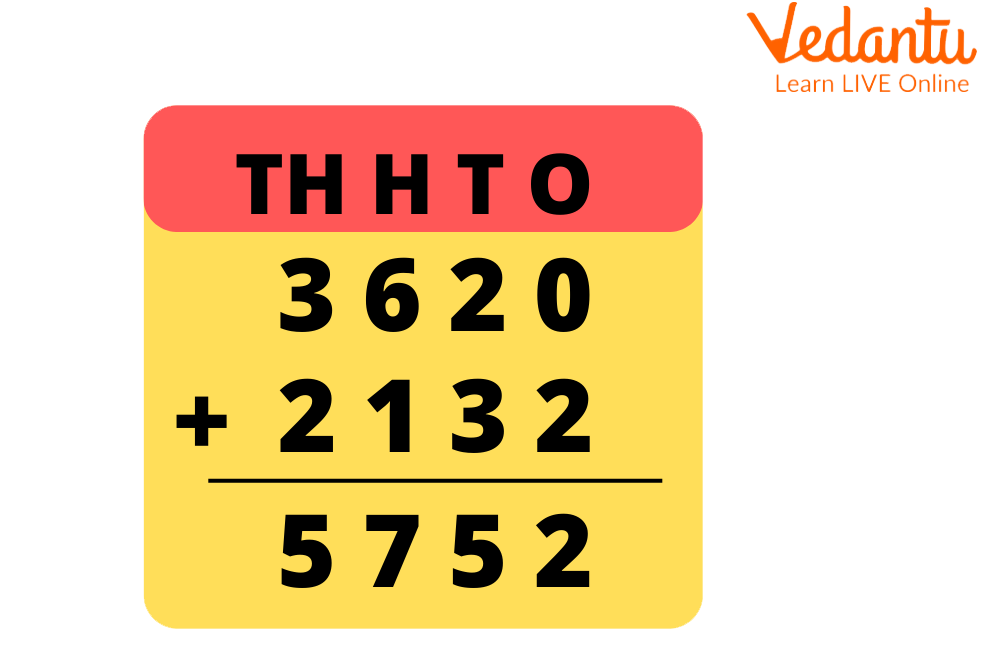Sum of 3620 and 2132

No. of males and women in the village $\begin{array}{l} = 3620 + 2132\\ = 5752\end{array}$

Therefore, the total population of the village is 5752.

Example 2: There are 5228 books on math, 2360 books on science, and 1921 books on English literature in a library. The library contains how many books?

Solution:

No. of math books = 5228, no. of science = 2360 and no. of English literature books = 1921.

According to the given question,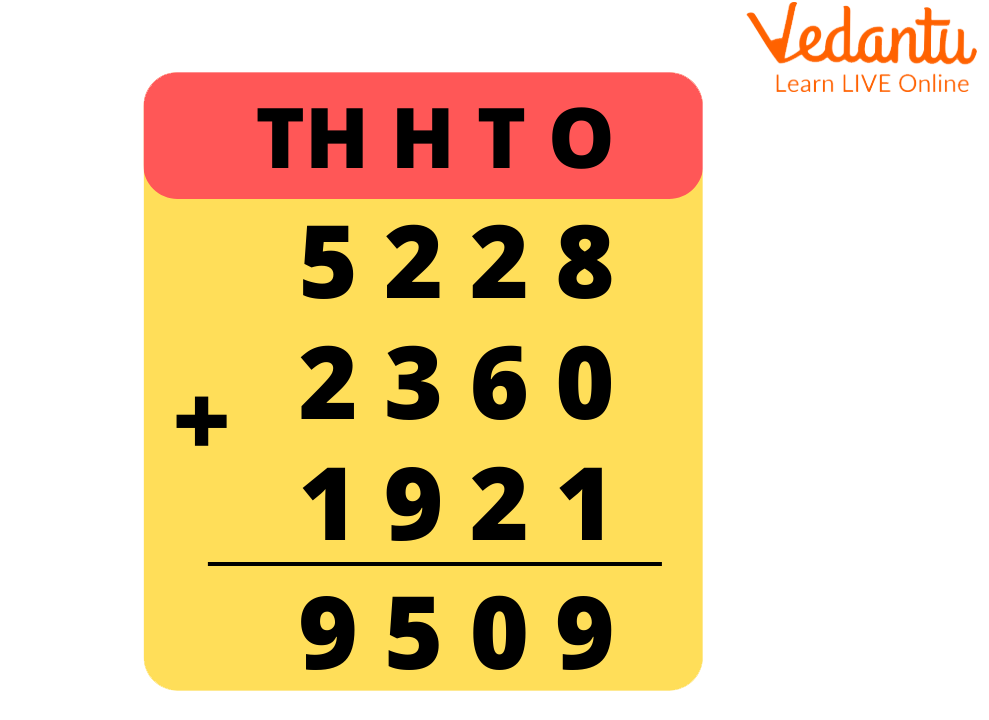Sum of 5228, 2360, and 1921

Total no. of books $\begin{array}{l} = 5228 + 2360 + 1921\\ = 9509\end{array}$

Therefore, the total number of books in the library is 9509.

Example 3: In 2015, a clockmaker bought 7,969 clocks. He bought 1,514 more clocks in 2016. What is the total number of clocks?

Solution:

No. of clocks that were bought in 2015 = 7,969, and no. of clocks that were bought in 2016 = 1,514

According to the given question,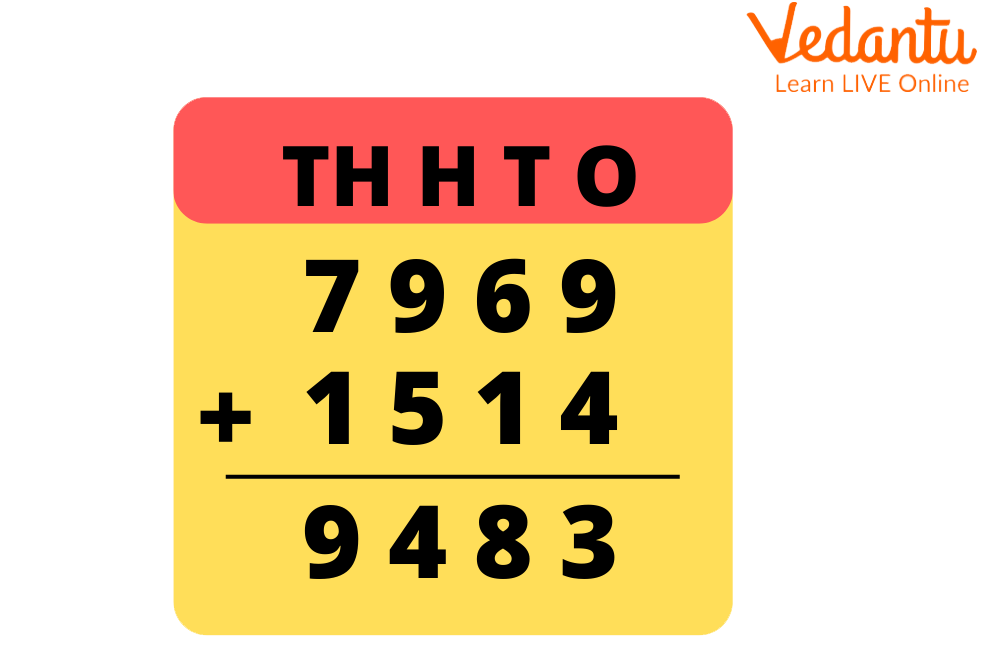Sum of 7969 and 1514

Total no. of clocks $\begin{array}{l} = 7,969 + 1,514\\ = 9,483\end{array}$

Therefore, the total number of clocks bought in these two years is 9,483.

## Worksheet on 4-Digit Subtraction:

Now, let us see a look at some 4 Digit Subtraction worksheets:

Example 1: 2300 children attend a school. How many boys are there if 1238 are girls?

Solution:

Total no. of children = 2300 and no. of girls = 1238

According to the given question,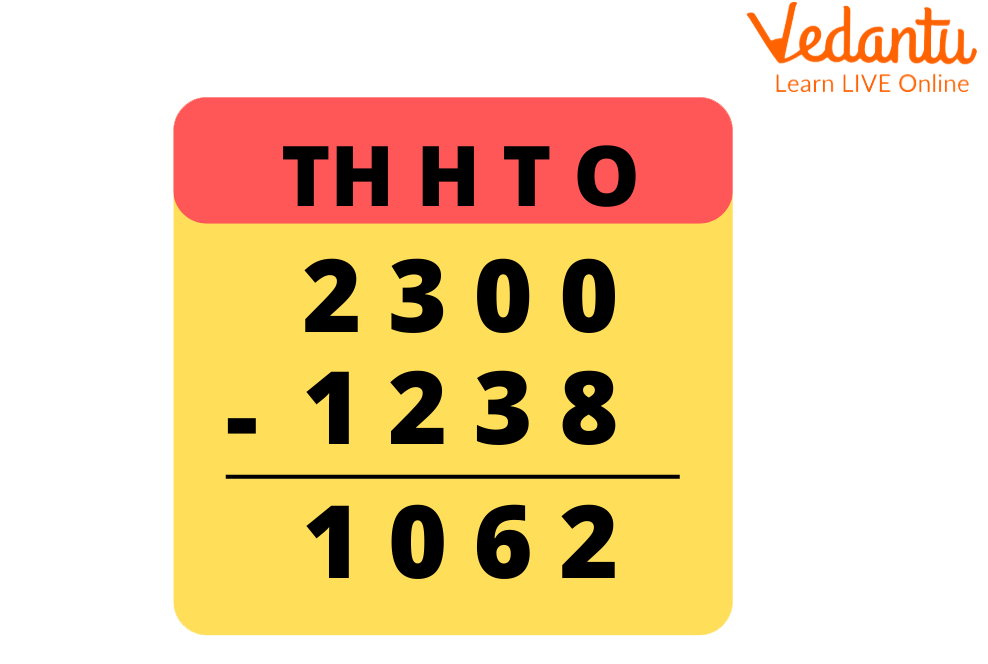Difference between 2300 and 1238

No. of boys $\begin{array}{l} = 2300 - 1238\\ = 1062\end{array}$

Therefore, the total no. of boys in the school is 1062.

Example 2: How many eggs were left after a restaurant used 1,657 of the 8,121 eggs they bought for the month?

Solution:

Total no. of eggs bought = 8,121, and no. of eggs used = 1,657.

According to the given question,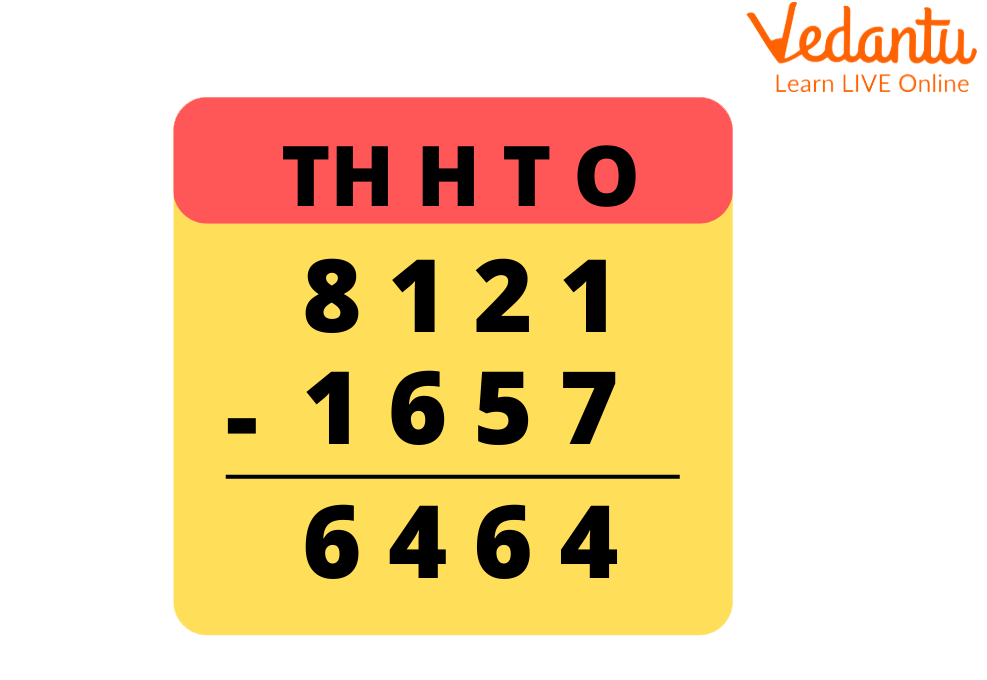Difference between 8121 and 1657

No. of eggs left unused $\begin{array}{l} = 8,121 - 1,657\\ = {\rm{6,464}}\end{array}$

Therefore, the number of eggs left in the restaurant is 6,464.

Example 3: Bryan scored 4,743 , while Matthew scored 2,687 in a video game. How many additional points did Matthew get?

Solution:

Score of Bryan = 4,743 and score of Matthew = 2,687

According to the given question,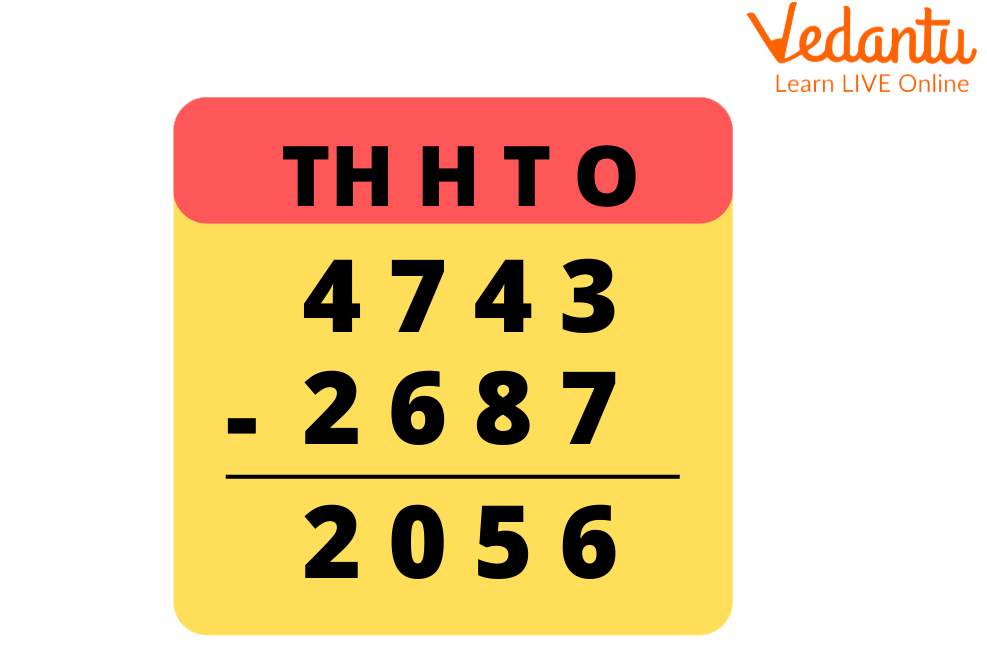Difference between 4743 and 2687

Extra points for Matthew $=4,743-2,687=2,056$

Therefore, the additional point that Matthew got is 2,056.

### Conclusion:

We learn addition and subtraction at a very young age. After learning about numbers, we learn how to count with them. As counting increases the number, it is somewhere very closely related to addition. In contrast, we learn about subtraction by counting how many candies we still have after eating.

4-digit addition and subtraction include addition and subtraction of two or more numbers, each of four digits.

## FAQs on Word Problems on 4 Digit Addition and Subtraction

1: Why do kids find arithmetic word problems challenging?

Mathematical word problems frequently present a difficulty since they call for students to read and understand the problem's text, recognise the question that needs to be answered, and then develop and solve a numerical solution.

2: Why is it crucial to solving word problems?

Because they demonstrate a thorough understanding of the math being taught, word problems are an important tool for teachers using testing. Additionally, they highlight for parents and teachers the areas where students are having trouble connecting.

3: Why is adding so important to daily life?

Setting the table, getting change at the store, and participating in some games are just a few examples of how adding and subtracting can be useful in daily life. Children learn about addition and subtraction to prepare them for learning about multiplication and division in school.